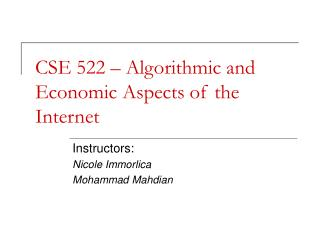Download PresentationCSE 522 – Algorithmic and Economic Aspects of the InternetCSE 522 – Algorithmic and Economic Aspects of the Internet - PowerPoint PPT Presentation

Download PresentationCSE 522 – Algorithmic and Economic Aspects of the Internet
An Image/Link below is provided (as is) to download presentation

Download Policy: Content on the Website is provided to you AS IS for your information and personal use and may not be sold / licensed / shared on other websites without getting consent from its author. While downloading, if for some reason you are not able to download a presentation, the publisher may have deleted the file from their server.

- - - - - - - - - - - - - - - - - - - - - - - - - - - E N D - - - - - - - - - - - - - - - - - - - - - - - - - - -
Presentation Transcript

1. CSE 522 – Algorithmic and Economic Aspects of the Internet Instructors: Nicole Immorlica Mohammad Mahdian

2. This lecture Probabilistic generative models for social networks (in particular web graph)

3. Why look for generative models? • Designing and testing algorithms for the web • E.g.: • Compressing the web graph • Designing crawling strategies • Search algorithms on P2P networks • … • Explaining why web has certain properties • For example, the central limit theorem tells us why we often see the Gaussian distribution in practice. • Is there a similar explanation for the power law distribution? • Predicting what “might” happen in the future • E.g.: An AIDS epidemic? An Internet black out? A residential segregation?

4. Characteristics of a good model • Simple • Plausible • Exihibits the observed properties • Power law • Small world • Locally dense, globally sparse

5. Power law distribution • From last lecture: power laws everywhere! • Income distribution (Pareto 1896) • Word frequencies (Estoup 1916, Zipf 1932) • City population (Auerbach 1913, Zipf 1949) • Scientific productivity (Lotka 1926) • Internet graph degree dist (FFF 1999) • Web graph degree dist (BKMRRSTW 2000) • Dist. of file sizes • … • Why?

6. Models and explanations for power law • Optimization (“power law is the best design”) • Mandelbrot 1953: Zipf’s law is the most efficient design. • Carlson & Doyle 1999, Fabrikant et al. 2002 (HOT) • Monkeys typing randomly • Miller 1957: even a monkey typing randomly can generate a power law. • Multiplicative processes & Log-normal dist. • Gibrat 1930, Champernowne 1955, Gabaix 1999 • Preferential growth (“the rich get richer”) • Simon 1955, Yule 1925

7. Log-normal distribution • Central limit Thm: Product of many indep. distributions is approximately log-normal.

8. Multiplicative process and power law • Multiplicative processes can sometimes generate power law instead of log-normal: • Multiplicative process with a minimum Chambernowne 1953, Gabaix 1999 • Random stopping time Montroll and Schlesinger 1982,1983

9. Preferential growth • The system “grows”. • The probability of a new member joining a group is proportional to its current size. Simon 1955, Yule 1925 (for biological systems) Barabasi and Albert 1999: preferential attachment for web graph

10. Random graph models • Erdos-Renyi random graphs G(n,p) • n vertices, there is an edge between each pair independently with probability p. • G(n,p) at a glance: • Average degree np. Binomial degree dist. • p < 1/n: union of small simple connected comp. • p > 1/n: a “giant” complex component emerges (still many small connected components) • p > ln(n)/n: connected.

11. The ACL model • Proposed by Aiello, Chung, and Lu, 2000. • Fix a degree sequence d (e.g., power law). • Put di copies of the i’th vertex. • Pick a random matching. • Contract the di copies of the i’th vertex • Essentially a variant of G(n,p), with the degree distribution explicitly enforced.

12. Preferential attachment • Start with a graph with one node. • Vertices arrive one by one. • When a vertex arrives, it connects itself to one (m, in general) of the previous vertices, with probability proportional to their degrees.

13. Preferential attachment • Heuristic analysis (Barabasi-Albert): degree distribution follows a power law with exponent -3. • Theorem (Bollobas, Riordan, Spencer, Tusnady). For d < n1/16, the fraction of vertices that have degree d is almost surely around